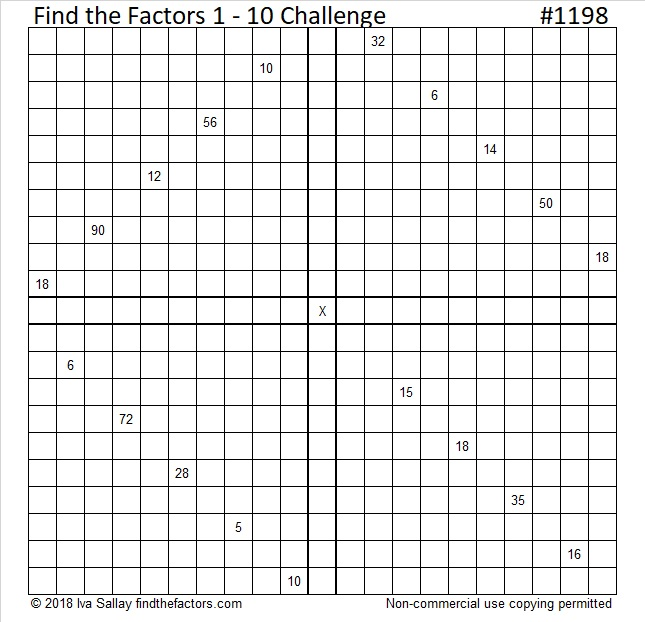# 1198 Challenge Puzzle

You can solve this Find the Factors 1 – 10 puzzle if you use logic. Guessing and checking will likely only frustrate you. Go ahead and give logic a try!Print the puzzles or type the solution in this excel file: 12 factors 1187-1198

Now I’ll share some facts about the number 1198:

• 1198 is a composite number.
• Prime factorization: 1198 = 2 × 599
• The exponents in the prime factorization are 1 and 1. Adding one to each and multiplying we get (1 + 1)(1 + 1) = 2 × 2 = 4. Therefore 1198 has exactly 4 factors.
• Factors of 1198: 1, 2, 599, 1198
• Factor pairs: 1198 = 1 × 1198 or 2 × 599
• 1198 has no square factors that allow its square root to be simplified. √1198 ≈ 34.612141198 is also palindrome 262 in BASE 23

This site uses Akismet to reduce spam. Learn how your comment data is processed.